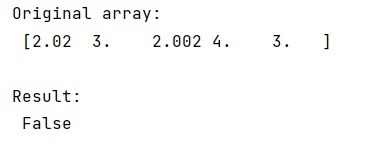# Function to determine if two numbers are nearly equal when rounded to n significant decimal digits

Learn about the function to determine if two numbers are nearly equal when rounded to n significant decimal digits.
Submitted by Pranit Sharma, on January 23, 2023

NumPy is an abbreviated form of Numerical Python. It is used for different types of scientific operations in python. Numpy is a vast library in python which is used for almost every kind of scientific or mathematical operation. It is itself an array which is a collection of various methods and functions for processing the arrays.

## Determining if two numbers are nearly equal when rounded to n significant decimal digits

Suppose we apply a round function on some values of a NumPy array and we need to check if two floating points are nearly equal or not. We need to determine a function that will return True if both values have the same values when rounded to a significant figure.
It is important that both the values must be of the same data type (int, float). Python math library provides a standard way of doing this.

The math library has an isclose() method which takes two values a and b and returns True if the values a and b are close to each other and False otherwise.

Let us understand with the help of an example,

## Python code to demonstrate the example of the function to determine if two numbers are nearly equal when rounded to n significant decimal digits

```# Import numpy
import numpy as np

# Import math
import math

# Creating a numpy array
arr = np.array([2.02, 3, 2.002, 4, 3])

# Display original array
print("Original array:\n",arr,"\n")

# Check if two values are equal
res = math.isclose(arr,arr)

# Display result
print("Result:\n",res,"\n")
```

Output: